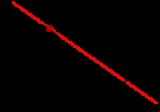xDerivativeWordNet

(1)   Resulting from or employing derivation
"A derivative process"
"A highly derivative prose style"

### noun

(2)   The result of mathematical differentiation; the instantaneous change of one quantity relative to another; df(x)/dx
(3)   (linguistics) a word that is derived from another word
"`electricity' is a derivative of `electric'"
(4)   A financial instrument whose value is based on another securityWiktionaryText

### Etymology

Middle English, from dérivatif and derivatus; see derive.

1. Imitative of the work of someone else.
2. Referring to a work, such as a translation or adaptation, based on another work that may be subject to copyright restrictions.
3. Having a value that depends on an underlying asset of variable value.
4. Lacking originality.

### Noun

1. Something derived.
2. A word that derives from another one.
3. A financial instrument whose value depends on the valuation of an underlying asset; such as a warrant, an option etc.
4. A chemical derived from another.
5. The derived function of a function.
The derivative of $f:f\left(x\right) = x^2$ is $f\text{'}:f\text{'}\left(x\right) = 2x$
6. The value of this function for a given value of its independent variable.
The derivative of $f\left(x\right) = x^2$ at x = 3 is $f\text{'}\left(3\right) = 2*3 = 6$.

#### Hyponyms

option, warrant, swap, convertible security, convertible, convertible bond, credit default swap, credit line note, financial futures contract, financial future, total return swap.